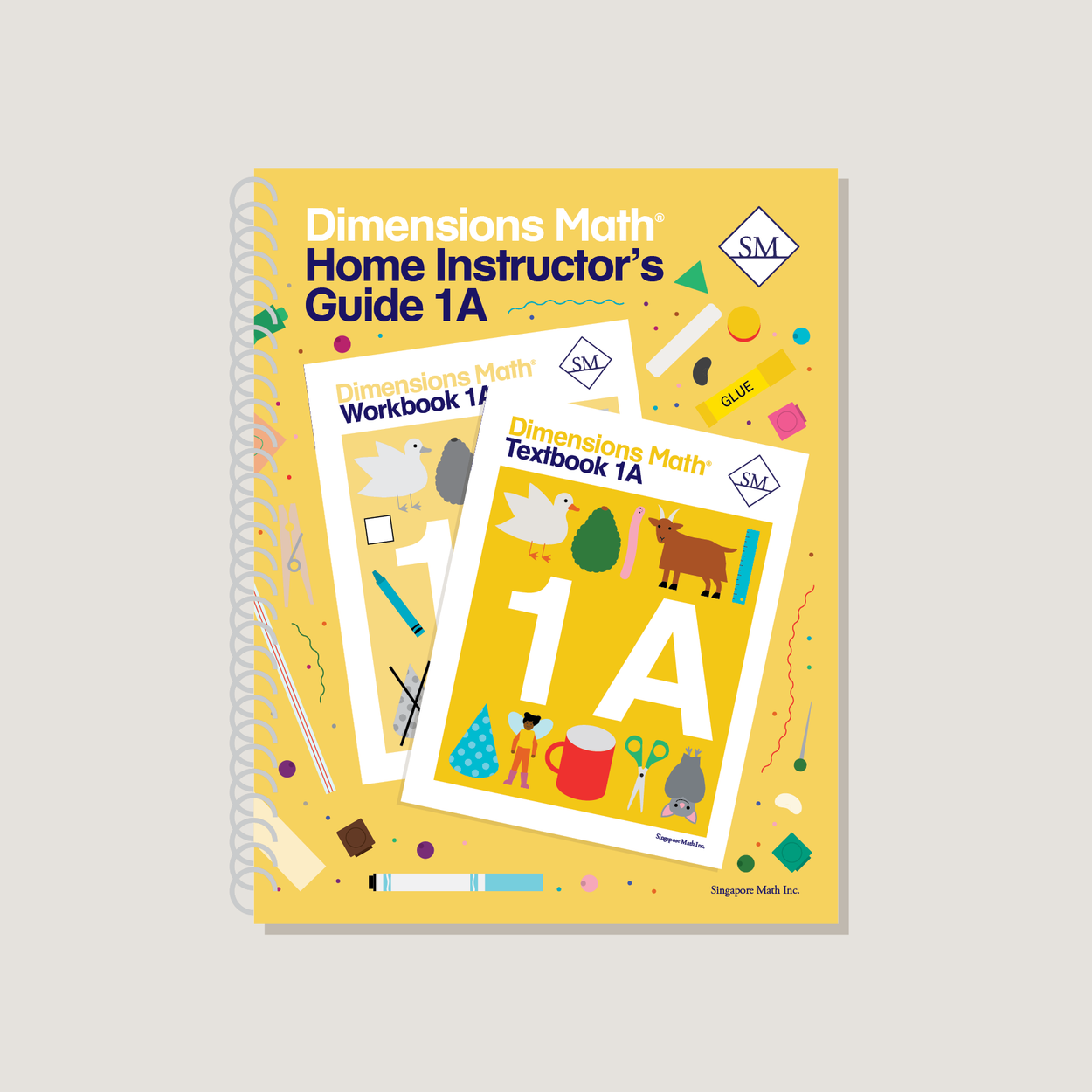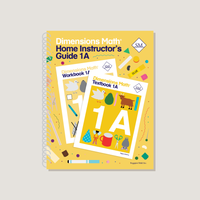# Dimensions Math Home Instructor's Guide 1A

\$20.00

Dimensions Math Home Instructor’s Guides are an essential resource for teaching Dimensions Math in a home or one-on-one setting. The purpose of the Guides is to help educators understand concepts in the Dimensions Math curriculum and teach as effectively as possible. They include extensive background notes, lesson suggestions, tips, and activities. Home Instructor’s Guides include answer keys for corresponding Dimensions Math Textbooks and Workbooks.

Note: Soft cover, spiral bound.

SKU: DMHIG1A

ISBN: 9781947226586

Pagecount: 144

Dimensions: 8.5 x 11 x 0.75 in

Weight: 1.1 lbs

Binding: Spiral

Color: Grayscale

Cover: Soft

Perforated: No

Sample Pages

Chapter 1: Numbers to 10
Lesson 1: Numbers to 10
Lesson 2: The Number 0
Lesson 3: Order Numbers
Lesson 4: Compare Numbers
Lesson 5: Practice

Chapter 2: Number Bonds
Lesson 1: Make 6
Lesson 2: Make 7
Lesson 3: Make 8
Lesson 4: Make 9
Lesson 5: Make 10 — Part 1
Lesson 6: Make 10 — Part 2
Lesson 7: Practice

Lesson 1: Addition as Putting Together
Lesson 4: Addition with Number Bonds
Lesson 5: Addition by Counting On
Lesson 8: Practice

Chapter 4: Subtraction
Lesson 1: Subtraction as Taking Away
Lesson 2: Subtraction as Taking Apart
Lesson 3: Subtraction by Counting Back
Lesson 4: Subtraction with 0
Lesson 5: Make Subtraction Stories
Lesson 6: Subtraction with Number Bonds
Lesson 8: Make Addition and Subtraction Story Problems
Lesson 9: Subtraction Facts
Lesson 10: Practice
Review 1

Chapter 5: Numbers to 20
Lesson 1: Numbers to 20
Lesson 2: Add or Subtract Tens or Ones
Lesson 3: Order Numbers to 20
Lesson 4: Compare Numbers to 20
Lesson 6: Subtraction
Lesson 7: Practice

Lesson 1: Add by Making 10 — Part 1
Lesson 2: Add by Making 10 — Part 2
Lesson 3: Add by Making 10 — Part 3
Lesson 4: Addition Facts to 20
Lesson 5: Practice

Chapter 7: Subtraction Within 20
Lesson 1: Subtract from 10 — Part 1
Lesson 2: Subtract from 10 — Part 2
Lesson 3: Subtract the Ones First
Lesson 4: Word Problems
Lesson 5: Subtraction Facts Within 20
Lesson 6: Practice

Chapter 8: Shapes
Lesson 1: Solid and Flat Shapes
Lesson 2: Grouping Shapes
Lesson 3: Making Shapes
Lesson 4: Practice

Chapter 9: Ordinal Numbers
Lesson 1: Naming Positions
Lesson 2: Word Problems
Lesson 3: Practice
Review 2

A & B Books: Our programs divide the school year into two semesters. “A” level books are for the first half of the school year. “B” level books are for the second half of the school year. You need both “A” and “B” material for a complete school year.

Required Components: Textbooks, Workbooks, and Guides (either Home Instructor’s Guides or Teacher’s Guides) are all necessary components. These three elements each serve a unique function and work together to build math mastery.

Printouts: Dimensions Math PK-5 Resources

Recommended Manipulatives: Dimensions Math Grades PK-5 Recommended Manipulatives